Home | | Maths 12th Std | Choose the Correct Answers

## Chapter: 12th Mathematics : UNIT 1 : Applications of Matrices and Determinants

Choose the Correct or the most suitable answer from the given four alternatives, Multiple choice questions with Answers, Solution and Explanation - Maths Book back 1 mark questions and answers with solution for Exercise Problems

Choose the Correct or the most suitable answer from the given four alternatives:

1. If | adj(adj A) |=|, then the order of the square matrix is

(1) 3

(2) 4

(3) 2

(4) 5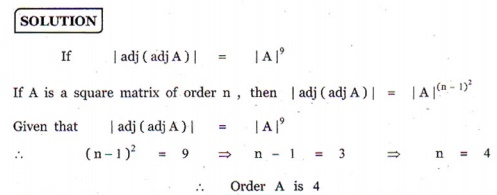2. If is a 3├Ś 3 non-singular matrix such that AA= Aand = AÔłĺA, then BB=

(1) A

(2) B

(3) I3

(4) BT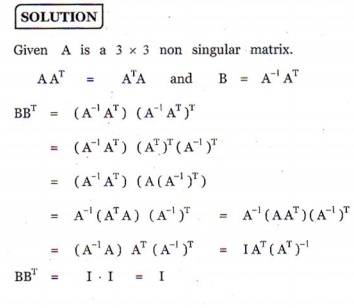3. If A =, B = adj A and C = 3, then | adj B | / |C| =

(1) 1/3

(2) 1/9

(3) 1/4

(4) 14. if, then A =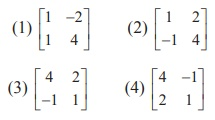Ans: (3)5. if, then 9I2 ÔÇô A =

(1) A-1

(2) A-1/2

(3) 3A-1

(4) 2A-16. if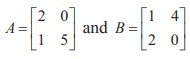then | adj ( AB) |=

(1) -40

(2) -80

(3) -60

(4) -207. If P=is the adjoint of 3├Ś 3 matrix and | |= 4 , then is

(1) 15

(2) 12

(3) 14

(4) 11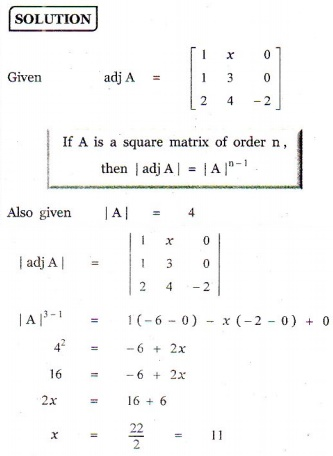8.If Aand AÔłĺthen the value of a23 is

(1) 0

(2) -2

(3) -3

(4) -19. If Aand are invertible matrices of some order, then which one of the following is not true?

(1) adj A =| A | A-1

(3) det A-1 = (det A)-1

(4) ( ABC)-1 = C-1B-1A-110. If ( AB)Ôłĺ, and A-1 =then BÔłĺ=Ans: (1)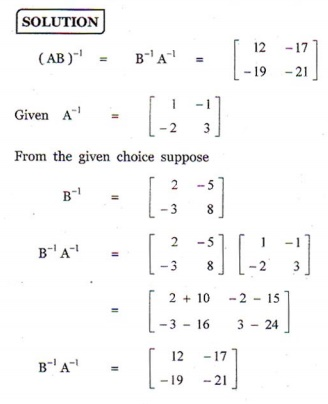11. If AAÔłĺis symmetric, then A=

(1) A-1

(2) (AT)2

(3) AT

(4) ( A-1 )212. If is a non-singular matrix such that AÔłĺ1, then ( A)Ôłĺ=Ans: (4)13.if A=and A= AÔłĺ, then the value of is

(1) -4/5

(2) -3/5

(3) 3/5

(4) 4/5

Ans: (1)14.if A=, and AB = I, then B=Ans: (2)15. if A=and A(adj A, then k=

(1) 0

(2) sin╬Ş

(3) cos╬Ş

(4) 1

Ans: (4)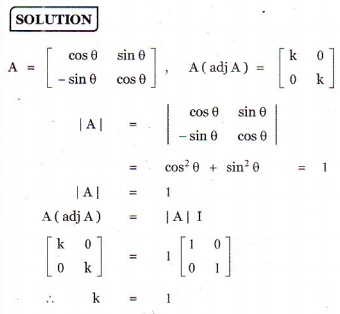16. If A=be such that ╬╗ AÔłĺ1 , then ╬╗ is

(1) 17

(2) 14

(3) 19

(4) 2117.If adj A=, adj B=, then adj ( AB) isAns: (2)18. The rank of the matrixis

(1) 1

(2) 2

(3) 4

(4) 3

Ans: (1)19. If xy= exy= ethen the values of and y are respectively,

(1) e(╬ö2 / ╬ö1 ) , e(╬ö3 / ╬ö1 )

(2) log(╬ö1/ ╬ö3 ), log(╬ö2/╬ö3)

(3) log(╬ö2/ ╬ö1 ), log(╬ö3/╬ö1)

(4) ) e(╬ö1 / ╬ö3 ) ,e(╬ö2/╬ö3 )20. Which of the following is/are correct?

(i) Adjoint of a symmetric matrix is also a symmetric matrix.

(ii) Adjoint of a diagonal matrix is also a diagonal matrix.

(iii) If A is a square matrix of order n and ╬╗ is a scalar, then adj( ╬╗ A) =  ╬╗n adj( A) .

(1) Only (i)

(2) (ii) and (iii)

(3) (iii) and (iv)

(4) (i), (ii) and (iv)21. If ¤ü A= ¤ü ([ B]) , then the system AX = of linear equations is

(1) consistent and has a unique solution

(2) consistent

(3) consistent and has infinitely many solution

(4) inconsistent22. If 0 ÔëĄ ╬Ş ÔëĄ ¤Ç and the system of equations + (sin╬Ş Ôłĺ (cos╬Ş )= 0, (cos╬Ş )Ôłĺ = 0, (sin╬Ş )Ôłĺ = 0 has a non-trivial solution then ╬Ş is

(1) 2¤Ç/3

(2) 3¤Ç/4

(3) 5¤Ç/6

(4) ¤Ç/4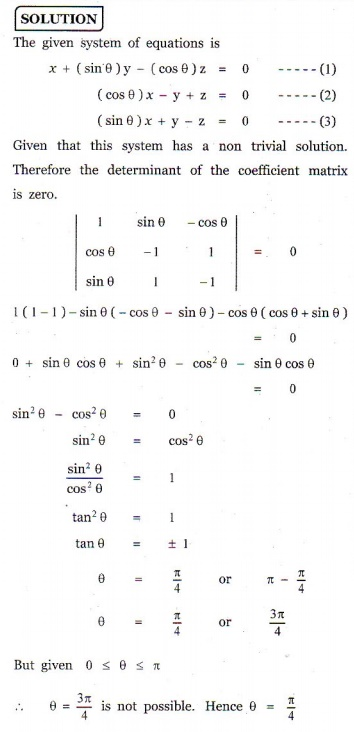23. The augmented matrix of a system of linear equations is. The system has infinitely many solutions if

(1) ╬╗ = 7, ╬╝ Ôëá -5

(2) ╬╗ = -7, ╬╝ = 5

(3) ╬╗ Ôëá 7, ╬╝ Ôëá -5

(4) ╬╗ = 7, ╬╝ = -524. let A =, and 4B =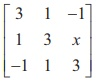, If is the inverse of , then the value of is

(1) 2

(2) 4

(3) 3

(4) 125.If A=, then adj(adj A) isAns: (1)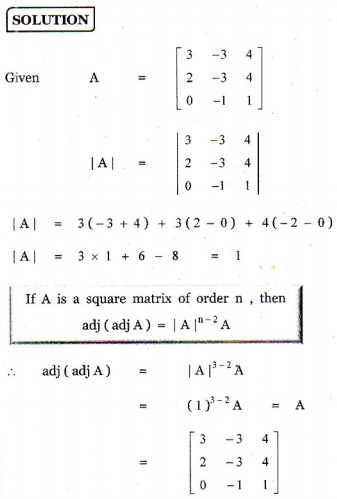Choose the Correct or the most suitable answer from the given four alternatives:

1. If | adj(adj A) |=|, then the order of the square matrix is

(1) 3

(2) 4

(3) 2

(4) 5

2. If is a 3├Ś 3 non-singular matrix such that AA= Aand = AÔłĺA, then BB=

(1) A

(2) B

(3) I3

(4) BT

3. If A =, B = adj A and C = 3, then | adj B | / |C| =

(1) 1/3

(2) 1/9

(3) 1/4

(4) 1

4. if, then A =Ans: (3)

5. if, then 9I2 ÔÇô A =

(1) A-1

(2) A-1/2

(3) 3A-1

(4) 2A-1

6. ifthen | adj ( AB) |=

(1) -40

(2) -80

(3) -60

(4) -20

7. If P=is the adjoint of 3├Ś 3 matrix and | |= 4 , then is

(1) 15

(2) 12

(3) 14

(4) 11

8.If Aand AÔłĺthen the value of a23 is

(1) 0

(2) -2

(3) -3

(4) -1

9. If Aand are invertible matrices of some order, then which one of the following is not true?

(1) adj A =| A | A-1

(3) det A-1 = (det A)-1

(4) ( ABC)-1 = C-1B-1A-1

10. If ( AB)Ôłĺ, and A-1 =then BÔłĺ=Ans: (1)

11. If AAÔłĺis symmetric, then A=

(1) A-1

(2) (AT)2

(3) AT

(4) ( A-1 )2

12. If is a non-singular matrix such that AÔłĺ1, then ( A)Ôłĺ=Ans: (4)

13.if A=and A= AÔłĺ, then the value of is

(1) -4/5

(2) -3/5

(3) 3/5

(4) 4/5

Ans: (1)

14.if A=, and AB = I, then B=Ans: (2)

15. if A=and A(adj A, then k=

(1) 0

(2) sin╬Ş

(3) cos╬Ş

(4) 1

Ans: (4)

16. If A=be such that ╬╗ AÔłĺ1 , then ╬╗ is

(1) 17

(2) 14

(3) 19

(4) 21

17.If adj A=, adj B=, then adj ( AB) isAns: (2)

18. The rank of the matrixis

(1) 1

(2) 2

(3) 4

(4) 3

Ans: (1)

19. If xy= exy= ethen the values of and y are respectively,

(1) e(╬ö2 / ╬ö1 ) , e(╬ö3 / ╬ö1 )

(2) log(╬ö1/ ╬ö3 ), log(╬ö2/╬ö3)

(3) log(╬ö2/ ╬ö1 ), log(╬ö3/╬ö1)

(4) ) e(╬ö1 / ╬ö3 ) ,e(╬ö2/╬ö3 )

20. Which of the following is/are correct?

(i) Adjoint of a symmetric matrix is also a symmetric matrix.

(ii) Adjoint of a diagonal matrix is also a diagonal matrix.

(iii) If A is a square matrix of order n and ╬╗ is a scalar, then adj( ╬╗ A) =  ╬╗n adj( A) .

(1) Only (i)

(2) (ii) and (iii)

(3) (iii) and (iv)

(4) (i), (ii) and (iv)

21. If ¤ü A= ¤ü ([ B]) , then the system AX = of linear equations is

(1) consistent and has a unique solution

(2) consistent

(3) consistent and has infinitely many solution

(4) inconsistent

22. If 0 ÔëĄ ╬Ş ÔëĄ ¤Ç and the system of equations + (sin╬Ş Ôłĺ (cos╬Ş )= 0, (cos╬Ş )Ôłĺ = 0, (sin╬Ş )Ôłĺ = 0 has a non-trivial solution then ╬Ş is

(1) 2¤Ç/3

(2) 3¤Ç/4

(3) 5¤Ç/6

(4) ¤Ç/4

23. The augmented matrix of a system of linear equations is. The system has infinitely many solutions if

(1) ╬╗ = 7, ╬╝ Ôëá -5

(2) ╬╗ = -7, ╬╝ = 5

(3) ╬╗ Ôëá 7, ╬╝ Ôëá -5

(4) ╬╗ = 7, ╬╝ = -5

24. let A =, and 4B =, If is the inverse of , then the value of is

(1) 2

(2) 4

(3) 3

(4) 1

25.If A=, then adj(adj A) isAns: (1)Tags : Applications of Matrices and Determinants , 12th Mathematics : UNIT 1 : Applications of Matrices and Determinants
Study Material, Lecturing Notes, Assignment, Reference, Wiki description explanation, brief detail
12th Mathematics : UNIT 1 : Applications of Matrices and Determinants : Choose the Correct Answers | Applications of Matrices and Determinants